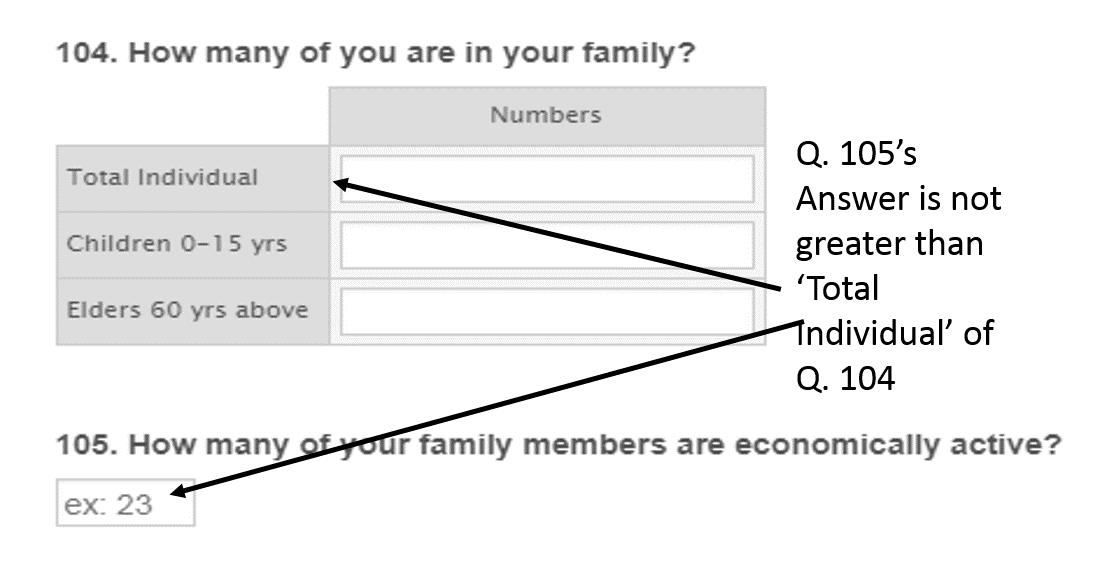# How to put condtion in an Input table to compare two fields?

•Aslam
Asked on April 20, 2019 at 03:55 AM

Sir,

I create a form, There is two questions.

1. How many members in your family?

2. How many of them earning money?

The second Question is depend on first and Answer of the second question's answer is equal or less than a number of first question's answer.

So, How to provide condition into second question based on first question's answer.

Need a help,

regards•roneet
Answered on April 20, 2019 at 04:58 AM

1. Add a Form Calculation widget to calculate the number of individuals.2. Add a text element to show the error message when the active member exceeds the total.

3. Add a condition to show the text and hide the submit button when the active member exceeds the total.Result:Feel free to test and clone this Form:

https://form.jotform.com/91092433684965

Thanks.

•Answered on April 20, 2019 at 05:56 AM

Sir,

Thanks,

In my survey, I could not want to add Calculation and Could not hide the next questions. In this situation what to do?

regards

•AndrewHag
Answered on April 20, 2019 at 06:35 AM

I couldn't see any hidden questions linked with that field. If I understood your question correctly, you don't want to use the Calculation widget and hide those fields?

If you don't want to use the Calculation widget then you can use Number field instead. You can avoid hiding the Number field by removing the Total individuals from the Input Tabel and creating a new field and label it as Total Members so that the users can directly enter the number in that field. And you can set the same condition given by my colleague in the previous solution.Coming to the error message, if you want to display the custom error message then the only way is you need to hide that field. Is there any other action that you wish to perform instead of displaying the error message so that we can provide the solution accordingly?